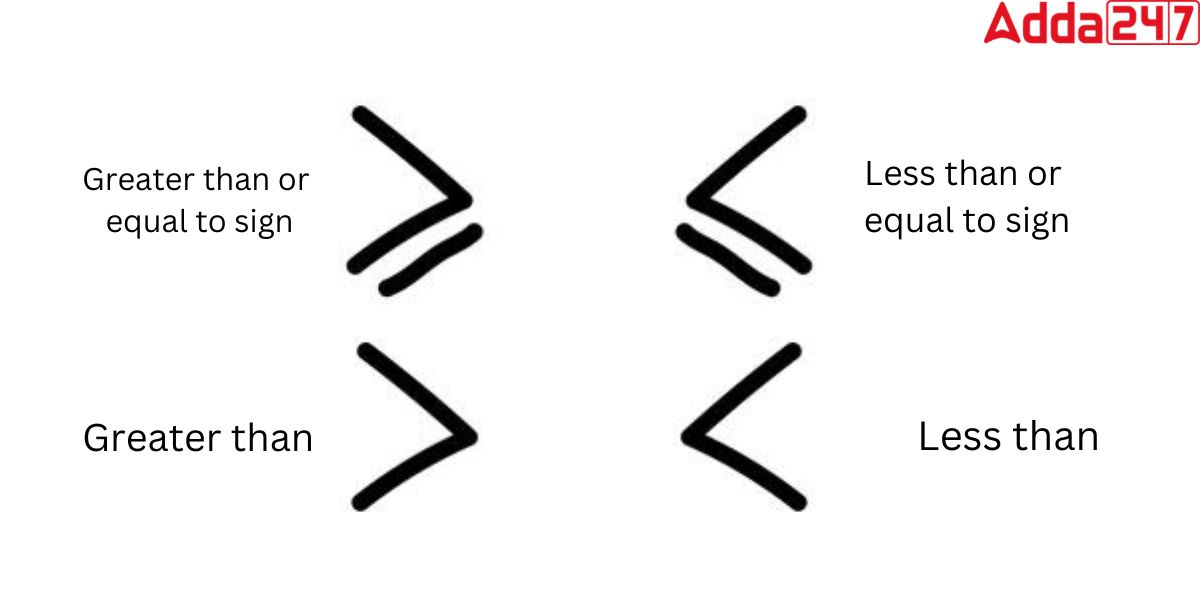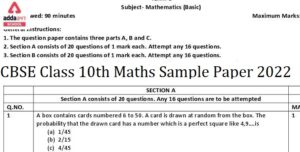Online Tution   »   Important Question   »   Greater than and Less than Symbols

# Greater Than and Less Than Symbols

## Greater Than and Less Than Symbols in Maths

Greater than and Less than Symbols: Nowadays, many students try to understand the basic concept of modern mathematics like Greater than and Less than Symbols, but the standard books are pretty harsh on them to be understood on it. That is why student uses technology and websites to understand the concept of it. If you are also here to understand the basic concept of Greater than and Less than Signs, then you are at the right place.

Please be with us till the end of this article, because Today, we will tell you every possible simplest way to tell you about the Greater than and Less than Symbols in mathematics.

## Greater Than and Less Than Symbols Meaning

The Meaning of the Greater Than and Less Than Symbols in Maths is exactly the opposite or vice-versa. Although You may assume the meaning of these Greater Than and Less Than signs by looking at their symbols. (>,<). The definition of Greater Than and Less Than Symbols explained in detail with the example below

## What is the definition of greater than symbol?

The greater than symbol (>), also called the “greater than sign”, is a mathematical symbol that is used to compare two values. The symbol looks like a small arrow pointing to the right and is usually used between two numbers, like this: 3 > 2.

This means that the first number (3) is greater than the second number (2). When two values are compared using the greater than symbol, the result is either TRUE or FALSE.

If the first value is greater than the second value, the result is TRUE. If the first value is not greater than the second value, the result is FALSE.

## What is the definition of less than symbol?

It is just opposite of the greater than symbol. The less than symbol (<) is a mathematical operator that indicates that one value is less than another value. It is typically used when comparing two numbers. For example, the following inequality shows that 5 is less than 10:

5 < 10

The less than symbol can also be used to compare other values, such as variables or expressions. For example, the following inequality shows that x is less than y:

x < y

## Pictures of Greater than and Less than Symbols## Greater Than and Less Than Signs: Tricks to Remember

There are the three methods to remember greater than and less than sign.

1. Alligator Method
2. L Method
3. Points Method
• Alligator Method

The alligator method is a simple way to help students remember the difference between the greater than and less than signs. Here’s how it works:

As we all know crocodile always open mouth to eat more fishes so we consider > symbol as crocodile and numbers on both sides as fishes.When we put the alligator mouth in front of the numbers, we can see which number is larger and which number is smaller.

For example, if we put the alligator mouth in front of the numbers 5 and 3, we can see that 5 is greater than 3 because the alligator’s mouth is open wide.

For example, if we put the alligator mouth in front of the numbers 5 >3, we can see that 5 is greater than 3 because the alligator’s mouth is open wide.

• L Method

The L method is an often-used way to remember greater than (>) and less than (<) signs. To do this, imagine that the greater than sign looks like a “less than” sign lying on its side.

The less than sign can then be thought of as two backwards Ls with the top end being higher. You can also see the spelling of Less is starting from L. So you can remember the less than symbol by using L Method.

• Points Method

You can easily remember the Greater Than symbol and the Less than symbol by using the placement. Here we are telling you how to remember these symbols using point method.

Greater than Symbol

The symbol to the left, consisting of two points, is the greater than sign (>). It indicates that one value is larger than another.

Less than symbol

The left side of the symbol has one point and the right side has two, representing Less Than (<).

## List Greater Than and Less Than Symbols with Examples

This is a list of all of the mathematical symbols you might need for typesetting mathematics.

 Greater Than and Less Than Symbols Examples Symbol Description Symbol Notation Example Greater than sign > 8>5 Less than sign < 3<9 Equal to sign = 3=3

## Greater Than and Less Than Signs : Differences

Most of the candidates find difficulties while tackling problems with the greater than sign and the greater than or equal to sign and the less than sign and the less than or equal to sign. to overcome this problem they should understand the differences between those signs.

### Difference between the greater than sign and the greater than or equal to sign

Answer. The greater than sign (>) indicates that the first value is strictly greater than the second value, & the greater than or equal to sign (≥) indicates that the first value is either greater to the second value or equal to it.

### Difference between the less than sign and the less than or equal to sign

The less than sign (<) indicates that the first value is strictly less than the second value, while the less than or equal to sign (≤) indicates that the first value is less than or equal to the second value.

## Greater Than and Less Than Symbols Examples

These are the example of greater than and less than sign:

1. 2/3 < 1 – This means that two-thirds is less than one.
2. 5 + 2 > 6 – This means that five plus two is greater than six.
3. 3x – 5 > 10 – This means that three times x minus five is greater than ten.
4. 7y – 3 < 20 – This means that seven times y minus three is less than 20.
5. 2x < x + 5 – This means that two times x is less than x plus five.
6. 4z + 2 > 10 – This means that four times z plus two is greater than ten.
7. 1/2 < 3/4 – This means that one-half is less than three-fourths.
8. 8m > 24 – This means that eight times m is greater than 24.
9. 5x – 2 < 13 – This means that five times x minus two is less than 13.
10. 2a + 3b > 10 – This means that two times a plus three times b is greater than 10

Sharing is caring!

## FAQs

### What do the greater than and less than signs mean in mathematics?

The greater than and less than signs are mathematical symbols, and these are used to represent inequality between two values. The greater than sign (>), when placed between two values, indicates that the first value is larger than the second value. The less than sign (<) indicates that the first value is smaller than the second value.

### How do you know which side of the sign represents the larger number?

To know which side of the sign represents the larger number, you need to understand that the sign points towards the smaller number. For example, in the expression 5 > 3, the sign point towards the 3, indicating that 3 is smaller than 5.

### How do the greater than and less than signs relate to real-life situations?

It could be use to comparing the heights of two people, the taller person can be represented by the greater than sign and the shorter person by the less than sign.

### Can the greater than and less than signs be used to compare non-numeric values?

No, the greater than and less than signs cannot be used to compare non-numeric values.

### Can the greater than and less than signs be used to compare more than two numbers?

Yes, the greater than and less than signs can be used to compare more than two numbers by chaining the symbols together.

### How do you use the greater than and less than signs in inequalities?

The greater than and less than signs can be used in inequalities to represent a range of values that meet certain criteria.

### What is the significance of the equals sign in relation to the greater than and less than signs?

The equals sign (=) is used to indicate equality between two values.

### How can you use the greater than and less than signs to compare fractions?

To compare fractions using the greater than and less than signs, you need to convert the fractions to a common denominator and then compare the numerators. For example, 1/2 < 3/4, because both fractions have a common denominator of 4 and the numerator of 3/4, is greater than the numerator of 1/2.

### What is the difference between the less than sign and the less than or equal to sign?

The less than sign (<) indicates that the first value is strictly less than the second value, while the less than or equal to sign (≤) indicates that the first value is less than or equal to the second value.

### What is the difference between the greater than sign and the greater than or equal to sign?

The greater than sign (>) indicates that the first value is strictly greater than the second value, & the greater than or equal to sign (≥) indicates that the first value is either greater to  the second value or equal to it.

### TOPICS:

•Class 10 Maths Board Paper 2023 {Most Ex...
•CBSE Class 10th Maths Term 2 Sample Pape...